GATE  >  Chemistry- CY 2015 GATE Paper (Practice Test)

# Chemistry- CY 2015 GATE Paper (Practice Test) - GATE

Test Description

## 65 Questions MCQ Test GATE Past Year Papers for Practice (All Branches) - Chemistry- CY 2015 GATE Paper (Practice Test)

Chemistry- CY 2015 GATE Paper (Practice Test) for GATE 2023 is part of GATE Past Year Papers for Practice (All Branches) preparation. The Chemistry- CY 2015 GATE Paper (Practice Test) questions and answers have been prepared according to the GATE exam syllabus.The Chemistry- CY 2015 GATE Paper (Practice Test) MCQs are made for GATE 2023 Exam. Find important definitions, questions, notes, meanings, examples, exercises, MCQs and online tests for Chemistry- CY 2015 GATE Paper (Practice Test) below.
Solutions of Chemistry- CY 2015 GATE Paper (Practice Test) questions in English are available as part of our GATE Past Year Papers for Practice (All Branches) for GATE & Chemistry- CY 2015 GATE Paper (Practice Test) solutions in Hindi for GATE Past Year Papers for Practice (All Branches) course. Download more important topics, notes, lectures and mock test series for GATE Exam by signing up for free. Attempt Chemistry- CY 2015 GATE Paper (Practice Test) | 65 questions in 180 minutes | Mock test for GATE preparation | Free important questions MCQ to study GATE Past Year Papers for Practice (All Branches) for GATE Exam | Download free PDF with solutions
 1 Crore+ students have signed up on EduRev. Have you?
Chemistry- CY 2015 GATE Paper (Practice Test) - Question 1

### Q. 1 – Q. 5 carry one mark each. Q. Choose the most appropriate word from the optios given below to complete the following sentence. The principal presented the chief guest with a ________________, as token of appreciation.

Chemistry- CY 2015 GATE Paper (Practice Test) - Question 2

### Choose the appropriate word/ pharse, out of the four options given below, to complete the following sentence. Frogs_______

Chemistry- CY 2015 GATE Paper (Practice Test) - Question 3

### Choose the most similar in meaning to the given word. Educe

Chemistry- CY 2015 GATE Paper (Practice Test) - Question 4

Operators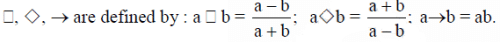Find the value of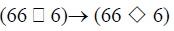Detailed Solution for Chemistry- CY 2015 GATE Paper (Practice Test) - Question 4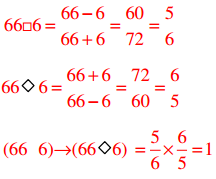Chemistry- CY 2015 GATE Paper (Practice Test) - Question 5

if logx(5/7) = -1/3, then the value of x is

Chemistry- CY 2015 GATE Paper (Practice Test) - Question 6

Q. 6 – Q. 10 carry Two mark each.

Q.

The following question presents a sentence, part of which is underlined. Beneath the sentence you find four ways of pharasing the underlined part. Following the requirements of the standard written english, select the answer that produces the most effective sentence.

Tuberculosis, together with its effect, "ranks one of the leading cause of death" in India

Chemistry- CY 2015 GATE Paper (Practice Test) - Question 7

Read the following paragraph and choose the correct statement.

climate change has reduced human security and threatened human well being. An ignored reality of human progress is that human securiy largely depends upon environmental security. But on the contrary, human progress seems contradictory to environmental security. To keep up both at the required level is a challange to be addressed by one and all. One of the ways to curb the climate change may be suitable scientific innovations, while the other way may be the Gadhian perspective on small scale progress wih focus on sustainability.

*Answer can only contain numeric values
Chemistry- CY 2015 GATE Paper (Practice Test) - Question 8

find the missing value.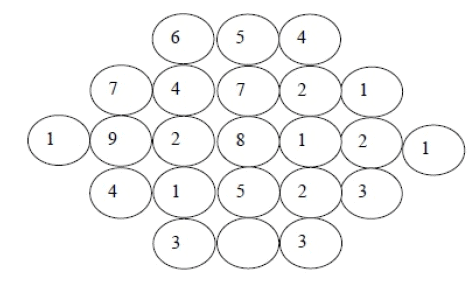Chemistry- CY 2015 GATE Paper (Practice Test) - Question 9

Acube of side 3 units is formed using a set of smaller cubes of side 1 unit. Find the proportion of the number of faces of the smaller cubes visible to those which are NOT visible.

Chemistry- CY 2015 GATE Paper (Practice Test) - Question 10

Hummpty Dumpty sits on a wall every day while having lunch. the wall sometimes breaks. A person sitting on the wall falls if the wall breaks.

Which one of the statements below is logically valid and can be inferred from te above sentences.?

Chemistry- CY 2015 GATE Paper (Practice Test) - Question 11

Q. 1 – Q. 25 carry one mark each

Q.

Which one of the following plots represents an acceptable wavefunction?

Chemistry- CY 2015 GATE Paper (Practice Test) - Question 12

When the operator, –h2d2/dx2, operates on the function eikx, the result is

Chemistry- CY 2015 GATE Paper (Practice Test) - Question 13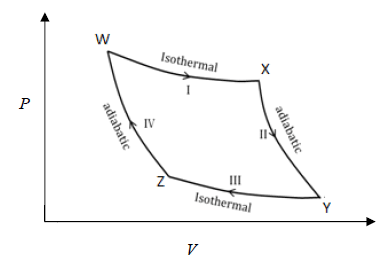From the above Carnot cycle undergone by an ideal gas, identify the processes in which the change in internal energy is NON-ZERO.

Chemistry- CY 2015 GATE Paper (Practice Test) - Question 14

For an ideal gas with molar mass M, the molar translational entropy at a given temperature is proportional to

Chemistry- CY 2015 GATE Paper (Practice Test) - Question 15

Which one of the following defines the absolute temperature of a system?

Chemistry- CY 2015 GATE Paper (Practice Test) - Question 16

Which of theollowing properties are characteristic of an ideal solution?

(i) (ΔmixG)T, P is negative

(ii) (ΔmixS)T, P is positive

(iii) (ΔmixV)T, P is positive

(iv) (ΔmixH)T, P is negative

Chemistry- CY 2015 GATE Paper (Practice Test) - Question 17

The expression for the equilibrium constant (Keq) for the enzyme catalyzed reaction given below, is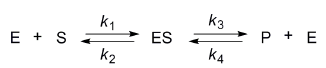*Answer can only contain numeric values
Chemistry- CY 2015 GATE Paper (Practice Test) - Question 18

Given the E0 values for the following reaction sequence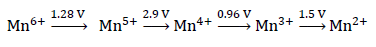the computed value of E0 for Mn6+→Mn2+ (in volts) is _______________

Chemistry- CY 2015 GATE Paper (Practice Test) - Question 19

The absorption spectrum of [Ti(H2O)6]3+ in solution comprises of a maximum with a shoulder. The reason for the shoulder is

Chemistry- CY 2015 GATE Paper (Practice Test) - Question 20

The ease of formation of the adduct, NH3·BX3 (where, X = F, Cl, Br) follows the order

Chemistry- CY 2015 GATE Paper (Practice Test) - Question 21

An efficient catalyst for hydrogenation of alkenes is [Rh(PPh3)3Cl]. However, [Ir(PPh3)3Cl] does not catalyze this reaction, because

Chemistry- CY 2015 GATE Paper (Practice Test) - Question 22

Among the given pH values, the O2 binding efficiency of hemoglobin is maximum at

Chemistry- CY 2015 GATE Paper (Practice Test) - Question 23

The intense red color of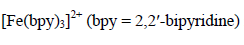is due to

Chemistry- CY 2015 GATE Paper (Practice Test) - Question 24

The compound with planar geometry is

Chemistry- CY 2015 GATE Paper (Practice Test) - Question 25

The electrical conductivity of a metal

Chemistry- CY 2015 GATE Paper (Practice Test) - Question 26

Which one of the following statements is INCORRECT?

Chemistry- CY 2015 GATE Paper (Practice Test) - Question 27

The absolute configuration of C2 and C3 in the following compound is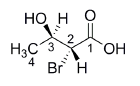Chemistry- CY 2015 GATE Paper (Practice Test) - Question 28

Among the following compounds, the one that is non-aromatic, is

Chemistry- CY 2015 GATE Paper (Practice Test) - Question 29

The correct order of reactivity of p-halonitrobenzenes in the following reaction is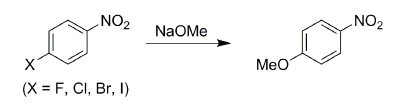Chemistry- CY 2015 GATE Paper (Practice Test) - Question 30

Tollen’s test is NEGATIVE for

Chemistry- CY 2015 GATE Paper (Practice Test) - Question 31

The compound given below is a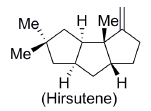Chemistry- CY 2015 GATE Paper (Practice Test) - Question 32

Amongst the following, the compound that DOES NOT act as a diene in Diels-Alder reaction is

Chemistry- CY 2015 GATE Paper (Practice Test) - Question 33

The following conversion is an example of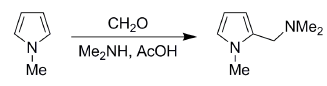Chemistry- CY 2015 GATE Paper (Practice Test) - Question 34

The mass spectrum of a dihalo compound shows peaks with relative intensities of 1:2:1 corresponding to M, M+2 and M+4 (M is the mass of the molecular ion), respectively. The compound is

*Answer can only contain numeric values
Chemistry- CY 2015 GATE Paper (Practice Test) - Question 35

Reaction of benzaldehyde and p-methylbenzaldehyde under McMurry coupling conditions (TiCl3 and LiAlH4) gives a mixture of alkenes. The number of alkenes formed is _______________

*Answer can only contain numeric values
Chemistry- CY 2015 GATE Paper (Practice Test) - Question 36

Q. 26 – Q. 55 carry two marks each.

Q.

The difference in the ground state energies (kJ/mol) of an electron in one-dimensional boxes of lengths 0.2 nm and 2 nm is _______________

*Answer can only contain numeric values
Chemistry- CY 2015 GATE Paper (Practice Test) - Question 37

The mean ionic activity coefficient of 0.001 molal ZnSO4 (aq) at 298 K according to the Debye- Hückel limiting law is (Debye-Hückel constant is 0.509 molal–½) _______________

Chemistry- CY 2015 GATE Paper (Practice Test) - Question 38

The process given below follows the Langmuir adsorption isotherm.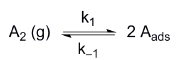If θ denotes the surface coverage and P denotes the pressure, the slope of the plot of 1/θ versus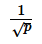is

*Answer can only contain numeric values
Chemistry- CY 2015 GATE Paper (Practice Test) - Question 39

For a gas phase unimolecular reaction at temperature 298 K, with a pre-exponential factor of 2.17 × 1013 s–1, the entropy of activation (J K–1 mol–1) is _______________

*Answer can only contain numeric values
Chemistry- CY 2015 GATE Paper (Practice Test) - Question 40

A liquid has vapor pressure of 2.02 ×103 N m–2 at 293 K and heat of vaporization of 41 kJ mol–1. The boiling point of the liquid (in Kelvin) is _______________

Chemistry- CY 2015 GATE Paper (Practice Test) - Question 41

The rotational partition function of a diatomic molecule with energy levels corresponding to J = 0 and 1, is (wher e, is a constant)

*Answer can only contain numeric values
Chemistry- CY 2015 GATE Paper (Practice Test) - Question 42

The internal energy of an i deal gas follows the equation U = 3.5 PV + k, where k is a constant. The gas expands fro m an initial volume of 0.25 m3 to a final volume of 0.86 m3. If the initial pressure is 5 N m–2, the change in internal energy (in Joules) is (given PV1.3 = constant) _______________

*Answer can only contain numeric values
Chemistry- CY 2015 GATE Paper (Practice Test) - Question 43

Th e solubility product of AgBr(s) is 5×10–13 at 298 K. If the standard reduction potential of the half-cell,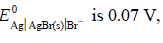, the standard reduction potential,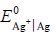(in volts) is _________.

*Answer can only contain numeric values
Chemistry- CY 2015 GATE Paper (Practice Test) - Question 44

One mole of a substance is heated from 300 K to 400 K at constant pressure. The  CP of the sub stance is given by cp(J-1mol-1)= 5+0.1 T. the change in entropy, in JK-1mol-1, of the substance is ______________

Chemistry- CY 2015 GATE Paper (Practice Test) - Question 45

h e potential energy (PE) versus reaction coordinate diagrams for electron transfer reactions with rate constants k1, k2 and k3, are given below. The increasing order of the rate constants is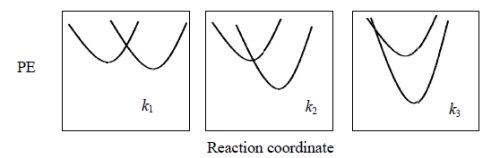Chemistry- CY 2015 GATE Paper (Practice Test) - Question 46

Th e distance b etween two successive (110) planes in a simple cubic lattice with lattice parameter

‘a’ is

Chemistry- CY 2015 GATE Paper (Practice Test) - Question 47

The percent transmittance of 8 × 10-5 M solution of KMnO4 is 39.8 when measured at 510 nm in a cell of path length of 1 cm. The absorbance and the molar extinction coefficient (in M-1cm-1) of this solution are, respectively,

Chemistry- CY 2015 GATE Paper (Practice Test) - Question 48

The value of ‘g’ and the number of signals observed for the reference standard, diphenylpicrylhydrazyl (DPPH), in the solid state ESR spectrum are, respectively

Chemistry- CY 2015 GATE Paper (Practice Test) - Question 49

Ammonolysis of S2Cl2 in an inert solvent gives

Chemistry- CY 2015 GATE Paper (Practice Test) - Question 50

The complexes K2[NiF6] and K3[CoF6] are

Chemistry- CY 2015 GATE Paper (Practice Test) - Question 51

The point group of IF7 is

Chemistry- CY 2015 GATE Paper (Practice Test) - Question 52

When one CO group is replaced by PPh3 in [Cr(CO)6], which one of the following statements is

TRUE?

Chemistry- CY 2015 GATE Paper (Practice Test) - Question 53

Identify X in the reaction, [Pt(NH3)4]2+ + 2 HCl X

Chemistry- CY 2015 GATE Paper (Practice Test) - Question 54

Identify the function of hemocyanin and the metal responsible for it.

*Answer can only contain numeric values
Chemistry- CY 2015 GATE Paper (Practice Test) - Question 55

The limiting current (in μA) from the reduction of 3 × 10-4 M Pb2+, using a dropping mercury electrode (DME) with characteristics, m = 3.0 mg s-1 and t = 3s, is (diffusion coefficient of Pb2+ = 1.2 × 10-5 cm2 s-1) _______________

*Answer can only contain numeric values
Chemistry- CY 2015 GATE Paper (Practice Test) - Question 56

The number of possible stereoisomers obtained in the following reaction is _______________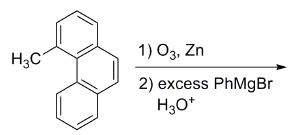Important : you should answer only the numeric value

Chemistry- CY 2015 GATE Paper (Practice Test) - Question 57

The major product formed in the following reaction is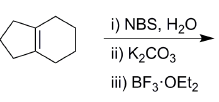Chemistry- CY 2015 GATE Paper (Practice Test) - Question 58

The most suitable reagent(s) to effect the following transformation is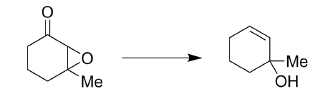Chemistry- CY 2015 GATE Paper (Practice Test) - Question 59

The major product formed in the following reaction is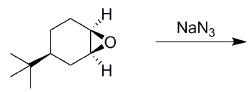Chemistry- CY 2015 GATE Paper (Practice Test) - Question 60

Solvolysis of the optically active compound X gives, mainly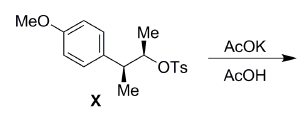Chemistry- CY 2015 GATE Paper (Practice Test) - Question 61

The major product formed in the following reaction is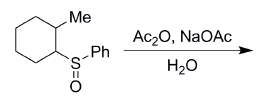Chemistry- CY 2015 GATE Paper (Practice Test) - Question 62

The tetrapeptide, Ala-Val-Phe-Met, on reaction with Sanger’s reagent, followed by hydrolysis gives

Chemistry- CY 2015 GATE Paper (Practice Test) - Question 63

The major product formed in the following reaction is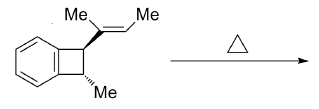Chemistry- CY 2015 GATE Paper (Practice Test) - Question 64

The Beckmann rearrangement of a bromoacetophenone oxime (C8H8BrNO) gives a major product having the following 1H NMR (δ, ppm): 9.89 (s, 1H), 7.88 (s, 1H), 7.45 (d, 1H, J = 7.2 Hz), 7.17 (m, 1H), 7.12 (d, 1H, J = 7.0 Hz), 2.06 (s, 3H). The structure of the product is

Chemistry- CY 2015 GATE Paper (Practice Test) - Question 65

The major products, K and L formed in the following reactions are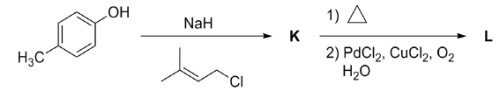## GATE Past Year Papers for Practice (All Branches)

407 docs|127 tests
Information about Chemistry- CY 2015 GATE Paper (Practice Test) Page
In this test you can find the Exam questions for Chemistry- CY 2015 GATE Paper (Practice Test) solved & explained in the simplest way possible. Besides giving Questions and answers for Chemistry- CY 2015 GATE Paper (Practice Test), EduRev gives you an ample number of Online tests for practice

## GATE Past Year Papers for Practice (All Branches)

407 docs|127 tests